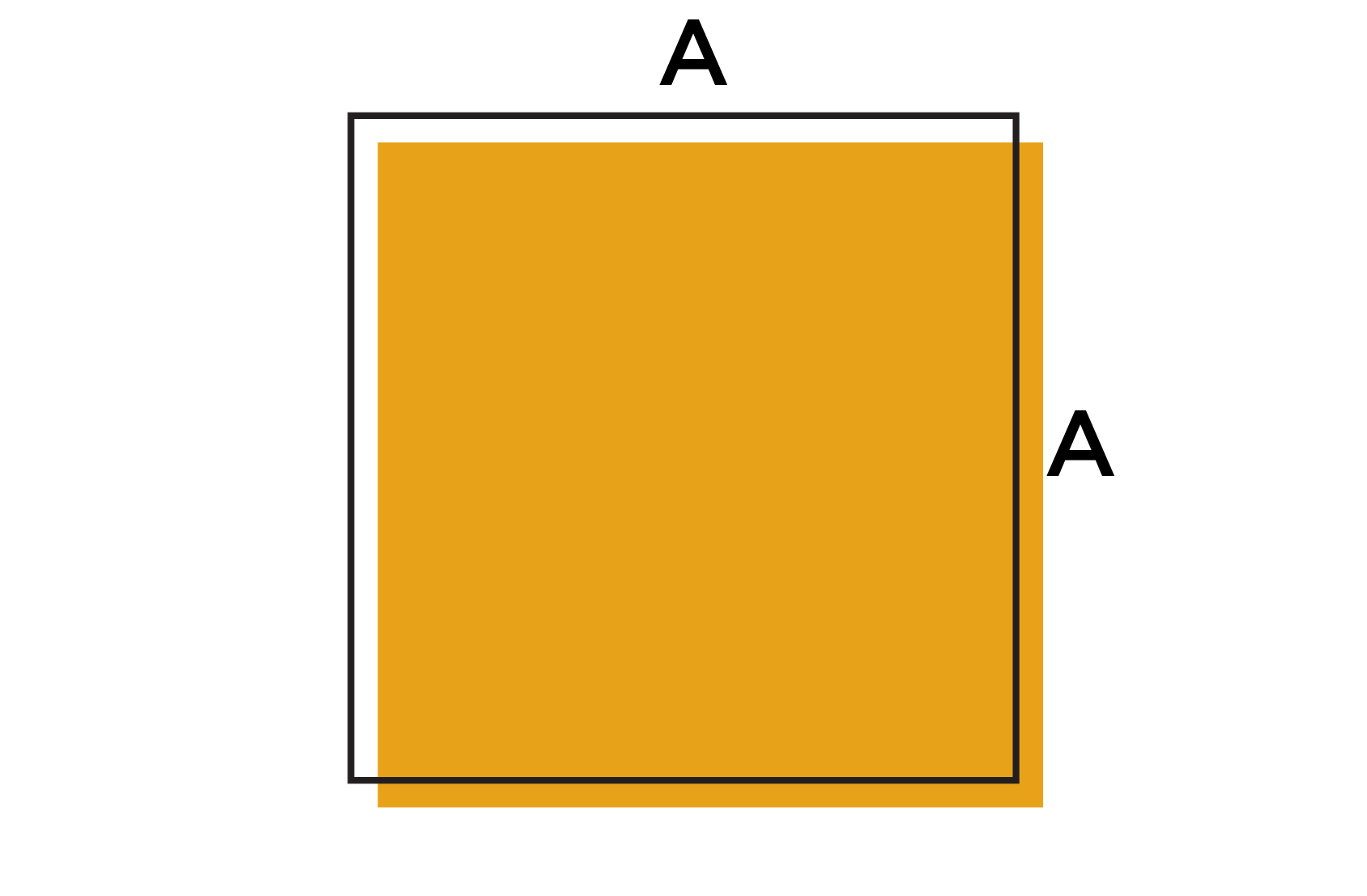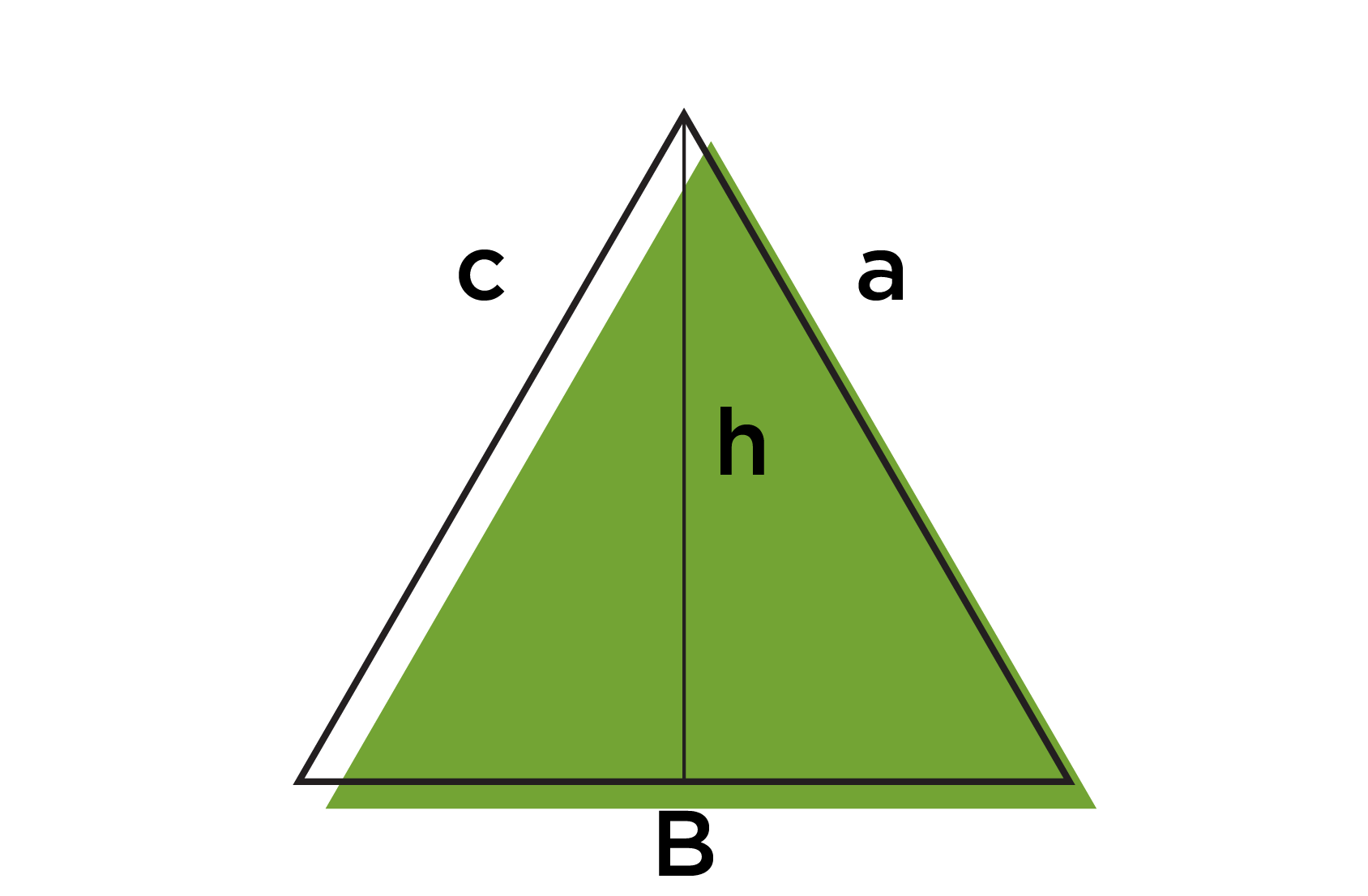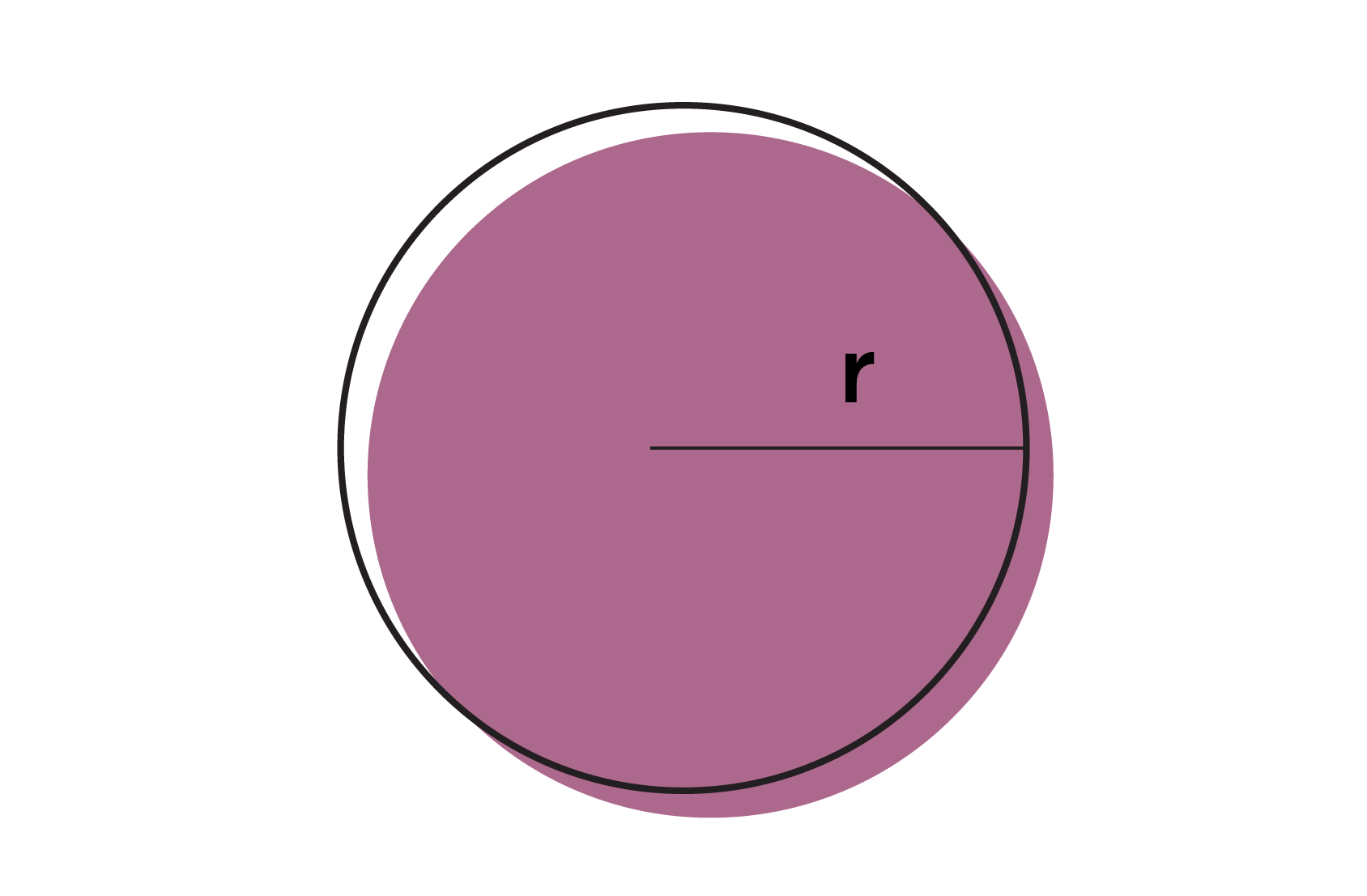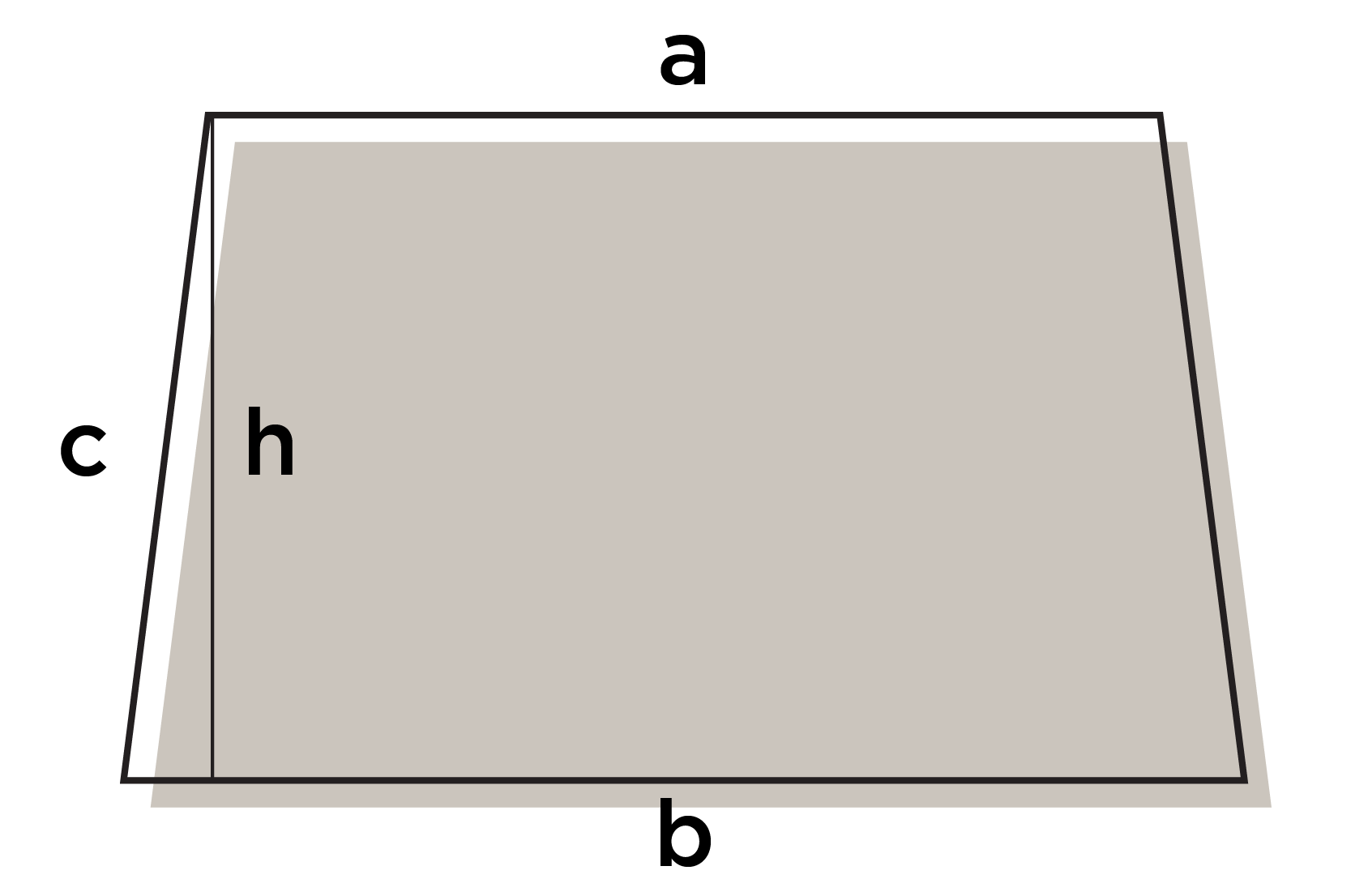The following sections provide resources to support trades students with the types of math problems they are likely to encounter in class and careers.

You can also check out the General Math section for more information and examples such as Arithmetic, Fractions, and Order of Operations.

The interactive workbooks linked in the table below were made specifically for trades students, by trades instructors. Each chapter provides video tutorials, practice questions with video analyses, practice tests, and step-by-step written examples. Each volume is an extremely valuable resource, no matter which way you prefer to learn!

## Trigonometry

Trigonometry is a branch of mathematics that deals with the relationships between the sides and angles of triangles.

The Pythagorean theorem is a mathematical concept that explains the relationship between the sides of a right triangle. A right triangle is a triangle that has one angle that measures exactly 90 degrees. If you have a right triangle with sides a, b, and c (where c is the hypotenuse), the Pythagorean theorem says that:

SOH CAH TOA is a mnemonic device used to remember the basic trigonometric ratios for right triangles. The ratios are defined as follows:

Sine (sin): the ratio of the length of the side opposite an angle to the length of the hypotenuse (the longest side).

Cosine (cos): the ratio of the length of the adjacent side to the length of the hypotenuse.

Tangent (tan): the ratio of the length of the opposite side to the length of the adjacent side.

To remember these ratios, you can use the following:

• SOH: Sine is Opposite over Hypotenuse
• CAH: Cosine is Adjacent over Hypotenuse
• TOA: Tangent is Opposite over Adjacent

## Transposing Equations

Transposing equations, also known as solving equations for a specific variable, is the process of rearranging an equation to isolate a particular variable on one side of the equation.

Equations typically involve one or more variables and mathematical operations such as addition, subtraction, multiplication, and division. When transposing equations, the goal is to manipulate the equation in such a way that the desired variable is separated, and all other terms are moved to one side.

To transpose an equation, you follow a series of steps to isolate the variable you want to solve for. Here’s a general overview of the process:

1. Identify the variable you want to solve for. Let’s call it “x.”
2. Move all other terms (constants and other variables) to the opposite side of the equation. Use inverse operations to undo any operations performed on the variable you’re transposing.
3. Continue simplifying the equation by applying the appropriate operations until you have the variable on one side and all other terms on the other side.
4. Check your solution by substituting the value you obtained for the variable back into the original equation. If the equation holds true, you have successfully transposed the equation.

It’s important to note that when transposing equations, you should perform the same operation on both sides of the equation to maintain equality. For example, if you add a value to one side of the equation, you must add the same value to the other side.

## Area and Perimeter

Area and perimeter are two fundamental concepts used to measure geometric figures such as polygons and circles. This Khan academy series covers how to calculate the area and perimeter for a variety of different shapes.

The area of a shape refers to the extent or size of its two-dimensional surface. It measures the amount of space enclosed within the boundaries of the shape. The unit of measurement for the area is typically square units, such as square centimeters (cm²) or square meters (m²).

The perimeter of a shape refers to the distance around its outer boundary. It measures the total length of the shape’s sides or curves. The unit of measurement for the perimeter is typically the same as the unit used for measuring the sides of the shape, such as centimeters (cm) or meters (m).

The table below provides a quick reference tool for the equations used to calculate the area and perimeter of common shapes.

Shape Area Perimeter
SquareArea = A x A or A² Perimeter = 4 x A
RectangleArea of a Rectangle

Area = L x W

Perimeter

Perimeter = 2 (L+W)

ParallelogramArea of a Parallelogram

Area = B x h

Here, B is the base, and h is the perpendicular height of the parallelogram.

Perimeter

Perimeter = 2 (B + a)

TriangleArea of a Triangle

Area = 1/2 (B x h)

Here, B is the base of the triangle (the entire length), and h is the height.

Perimeter

Perimeter = a + B + c

a² + b² = c²

CircleArea of a Circle

Area = π x r²

r = 1/2 diameter

Here, r is the radius of the circle.

Circumference

Circumference = 2πr

TrapezoidsArea of Trapezoids

Area = 1/2 (a + b) x h

Here, a and b are the lengths of the parallel sides, and h is the perpendicular height of the trapezoid.

Perimeter

Perimeter = a + b + 2c

Composites Area of Composite Shapes  Perimeter

## Surface Area and Volume

In mathematics, surface area and volume are measurements used to describe and quantify three-dimensional shapes.

Surface area (SA) refers to the total area occupied by the outer surfaces of a three-dimensional object. It represents the sum of the areas of all the faces, sides, and curved surfaces of the shape. Surface area is typically measured in square units, such as square centimeters (cm²) or square meters (m²).

For example, for a cube, the surface area can be calculated by adding up the areas of all six faces. If the length of each side of the cube is “a,” the formula for surface area (SA) is:

Similarly, for a cylinder, the surface area can be calculated by summing the areas of the two circular bases and the curved surface. The formula for the surface area (SA) of a cylinder with radius “r” and height “h” is:

Volume (V) refers to the amount of space enclosed by a three-dimensional object. It measures the capacity or total internal space occupied by the shape. Volume is typically measured in cubic units, such as cubic centimeters (cm³) or cubic meters (m³).

To calculate the volume of regular shapes such as a cube or rectangular prism, you can multiply the length, width, and height. For example, the volume (V) of a rectangular prism with length “L,” width “w,” and height “h” is given by:

The volume (V) of a sphere with radius “r” is:

And the volume (V) of a cylinder with a base radius “r” and height “h” is:

For more information on the formulas used to calculate the surface area and volume for a variety of different shapes, please take a look at the links below: